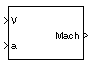# Mach Number

Compute Mach number using velocity and speed of sound

• Library:
• Aerospace Blockset / Flight Parameters

•## Description

The Mach Number block computes the Mach number. The Mach number is defined as

`$Mach=\frac{\sqrt{V\cdot V}}{a},$`

where a is the speed of sound and V is the velocity vector.

## Ports

### Input

expand all

Velocity vector, specified as an 3-element vector.

Data Types: `double`

Speed of sound, specified as a 1-by-1 array.

Data Types: `double`

### Output

expand all

Mach number, returned as a scalar.

Data Types: `double`

## Version History

Introduced before R2006a